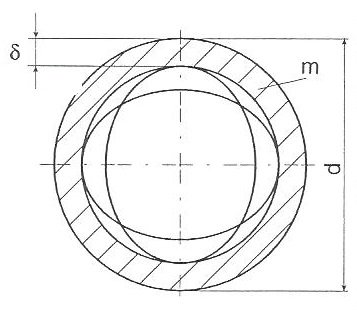# Mass Moments Inertia of Bodies

### Mass Moments Inertia of Bodies

Mass moments inertia (J) of bodies in Kgm²

#### Thin wall hollow cylinder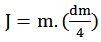where m = mass in Kg; dm = mean diameter in m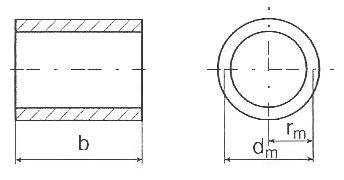#### Solid cylinder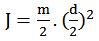where m = mass in Kg; d = diameter in m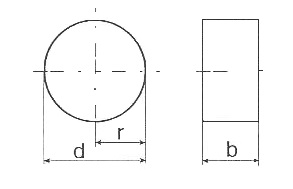#### Thick wall hollow cylinder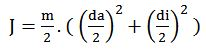where m = mass in Kg; da = outer diameter in m; di= inner diameter in m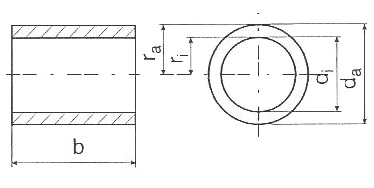#### Long thin bar with pivot point at center of gravity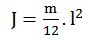where m = mass in Kg; l = Length of thin bar in m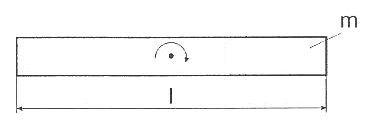#### Long thin bar with pivot point at end of bar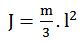where m = mass in Kg; l = Length of thin bar in m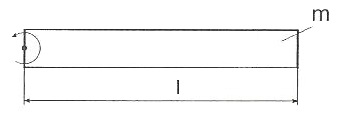#### Rectangular plate with pivot point at center of gravity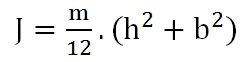where m = mass in Kg; h = breadth of rectangular plate in m; b = length of rectangular plate in m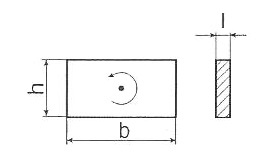#### Solid ball rotating at center of gravity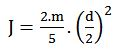where m = mass in Kg; d = diameter of solid ball in m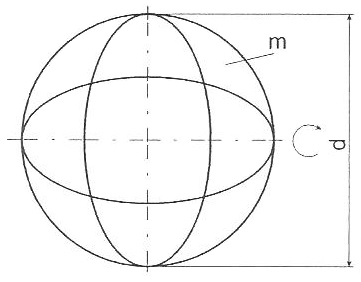#### Thin-walled ball shell rotating at center of gravity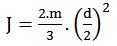where m = mass in Kg; d = outer diameter of thin-walled ball shell in m